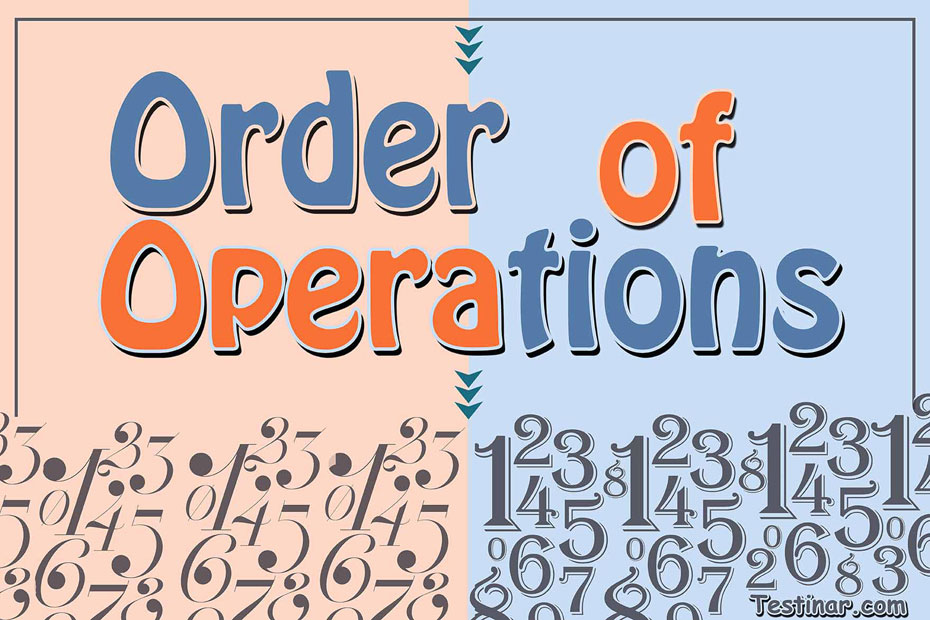## How to use order of operations

In mathematics, sometimes we are presented with a set of complex equations containing lot of operations. So, to sequentially and correctly solve the equation, we apply the order of operations. The order of operations tells the sequence in which the multiple operations in the mathematical expressions should be solved.
Now, to keep it simple, there is a short-form to remember the sequence of the order of operations. This is known as PEMDAS. Here each letter stands for:

• P = Parentheses
• E = Exponent
• M = Multiplication
• D = Division
• S = Subtraction

So, the PEMDAS rule dictates the sequences in which the multiple operations should be carried out.

• Parentheses- This is of the primary importance. Each and every group under the parenthesis should be solved first.
• Exponents- Next in sequence is all exponent operations. Work them out.
• Multiplication and Division- Now here, carefully divide or multiply each expression, moving from left to right.
• Addition and Subtraction- Similar to the multiplication and division part, carry out the addition or subtraction, moving from left to right.

#### Why should we follow the PEMDAS Rule?

The fundamental concept behind the discovery of PEMDAS rule was to let everyone conclude to the similar answer. Following any other sequence in the order of operations will lead you to a completely different and incorrect solution. Let’s look at what happens when we don’t follow PEMDAS rule.

Not Following Order of Operations: Here we just solve from left to right.
$$7 \times 2 + 6 \times (8 \div 4)$$
$$= 14 + 6 \times (8 \div 4)$$
$$= 20 \times (8 \div 4)$$
$$= 160 \div 4$$
$$= 40$$

Following Order of Operations: Here we apply PEMDAS rule.
$$7 \times 2 + 6 \times (8 \div 4)$$
$$= 7 \times 2 + 6 \times 2$$
$$= 14 + 6 \times 2$$
$$= 14 + 12$$
$$= 26$$
Now, we hope you understand why it is very important to apply PEMDAS rule in multiple operation expressions.

### Exercises for order of operations

1) $$9 \ \times \ 0 - 4$$ $$\ =$$

2) $$6 \ \times \ (-7) + 12$$ $$\ =$$

3) $$6 \ \times \ 4 - 12$$ $$\ =$$

4) $$3 \ \times \ (-8) + 5$$ $$\ =$$

5) $$7 \ \times \ 8 - 19$$ $$\ =$$

6) $$(-4) \ \times \ (-6) - 8$$ $$\ =$$

7) $$(-4) \ \times \ 6 + 3$$ $$\ =$$

8) $$4 \ \times \ 1 + 6$$ $$\ =$$

9) $$(-1) \ \times \ 4 - 6$$ $$\ =$$

10) $$16 \ \div \ 4 + 1$$ $$\ =$$

1) $$9 \ \times \ 0 - 4$$ $$\ = \color{red}{0 - 4 \ = -4}$$
2) $$6 \ \times \ (-7) + 12$$ $$\ = \color{red}{-42 + 12 \ = -30}$$
3) $$6 \ \times \ 4 - 12$$ $$\ = \color{red}{24 - 12 \ = 12}$$
4) $$3 \ \times \ (-8) + 5$$ $$\ = \color{red}{-24 + 5 \ = -19}$$
5) $$7 \ \times \ 8 - 19$$ $$\ = \color{red}{56 - 19 \ = 37}$$
6) $$(-4) \ \times \ (-6) - 8$$ $$\ = \color{red}{24 - 8 \ = 16}$$
7) $$(-4) \ \times \ 6 + 3$$ $$\ = \color{red}{-24 + 6 \ = -18}$$
8) $$4 \ \times \ 1 + 6$$ $$\ = \color{red}{4 + 12 \ = 16}$$
9) $$(-1) \ \times \ 4 - 6$$ $$\ = \color{red}{-4 - 6 \ = -10}$$
10) $$16 \ \div \ 4 + 1$$ $$\ = \color{red}{4 + 1 \ = 5}$$

## order of operations Quiz

### ISEE Middle Level Math Comprehensive Prep Bundle

$89.99$47.99

### CLEP College Math for Beginners 2022

$24.99$14.99

### ATI TEAS 6 Math in 10 Days

$20.99$19.99

### STAAR Grade 7 Math Comprehensive Prep Bundle

$104.99$36.99